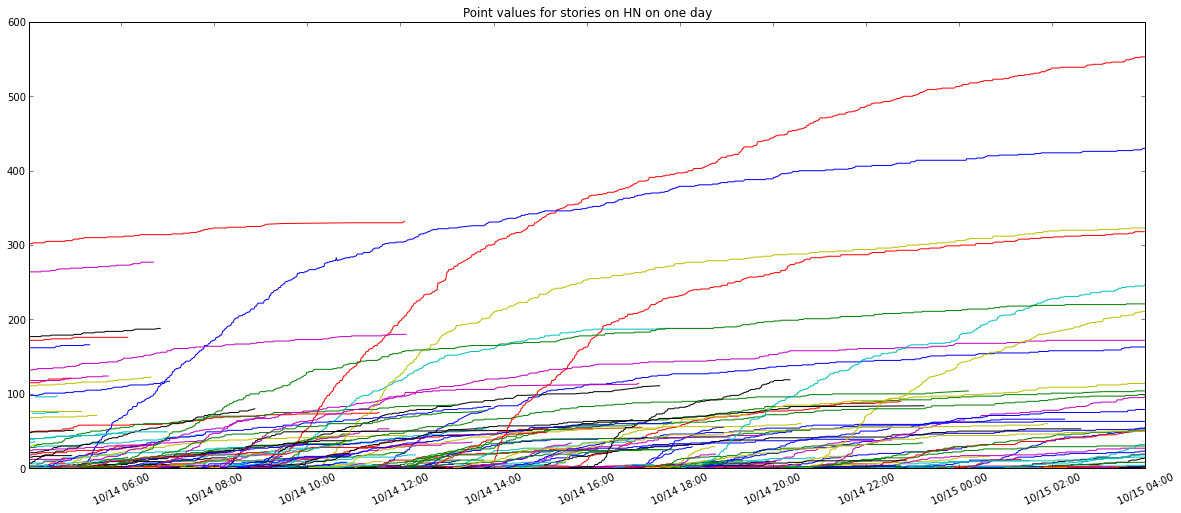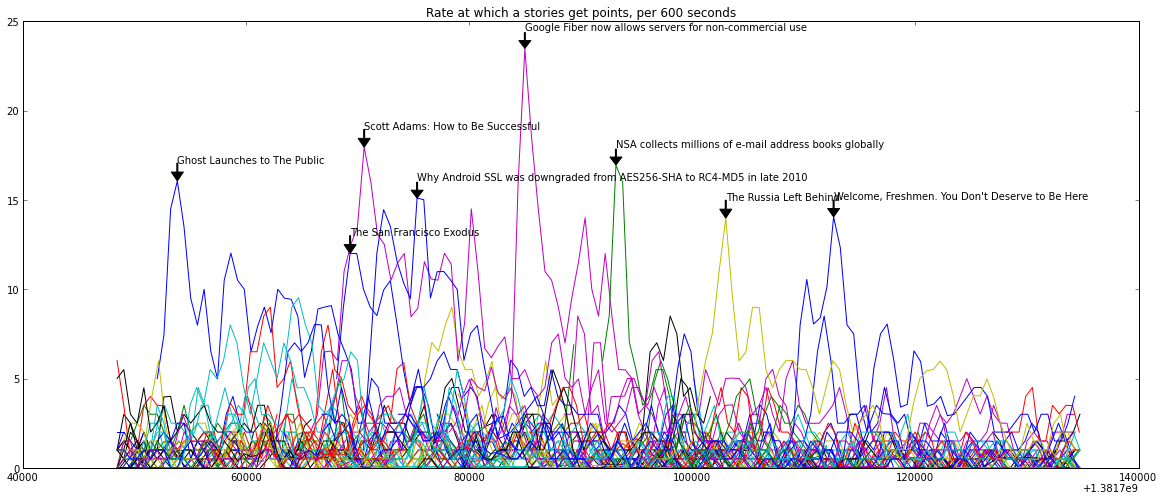In :
import glob
parsed = glob.glob('/home/karpathy/HNtrend/db/parsed_hn*.pgzip')
print 'total number of days of data: ', len(parsed)

total number of days of data:  47


In :
# lets get a sense of the data and what these records look like
import gzippickle
print parsed
print records['main']['posts']
print records['new']['posts']

/home/karpathy/HNtrend/db/parsed_hn_2013-10-15T04:00:10.971301.pgzip
{'domain': u'coffeepowered.net', 'title': u"No, Rails' CookieStore isn't broken", 'url': u'https://www.coffeepowered.net/2013/09/26/rails-session-cookies/', 'num_comments': 7, 'rank': 0, 'points': 26, 'user': u'thibaut_barrere', 'minutes_ago': -1, 'id': u'6546257'}
{'domain': u'archive.org', 'title': u'Backdoor found in D-Link Routers', 'url': u'http://web.archive.org/web/20131013143444/http://www.devttys0.com/2013/10/reverse-engineering-a-d-link-backdoor/', 'num_comments': 0, 'rank': 0, 'points': 1, 'user': u'DiabloD3', 'minutes_ago': 5, 'id': u'6546507'}


In :
print records['main']['posts']

{'domain': u'maverickblogging.com', 'title': u'CookieStore vulnerability in Rails 2.x, 3.x, 4.x.', 'url': u'http://maverickblogging.com/logout-is-broken-by-default-ruby-on-rails-web-applications/', 'num_comments': 33, 'rank': 1, 'points': 49, 'user': u'dgellow', 'minutes_ago': 180, 'id': u'6545923'}


In :
# lets visualize activity on one day. First compute activity for one day here
records = gzippickle.load(parsed) # one of the days

# this utility function will create dict of [post id] -> [(t, points), ...]
# where t is time
def record_to_activity(records):
activity = {}
titles = {}
for r in records:
m = r['main']['posts'] + r['new']['posts'] # lets put all posts together from both pages
t = int(r['time'].strftime("%s")) # seconds since epoch when this record was generated
pids_seen = []
for i,p in enumerate(m):
pid = p['id']
if pid in pids_seen: continue # there can be duplicates in NEW and FRONT
pids_seen.append(pid)
if not pid in activity: activity[pid] = []
L = activity[pid]
pts = p['points']
if pts > 0: # if its not positive we somehow had incorrect measurement
L.append((t, pts))
titles[pid] = p['title']

# make one more pass and sort all lists just to make sure time order is respected
for pid in activity: activity[pid].sort()
return (activity, titles)

activity, titles = record_to_activity(records)
print 'number of unique stories this day: ', len(activity)

number of unique stories this day:  893


In :
%matplotlib inline
import matplotlib.pyplot as plt
import matplotlib.dates
from datetime import datetime
import numpy as np
plt.rcParams['figure.figsize'] = (20.0, 8.0)

In :
def activity_to_plot(activity):
fig = plt.figure()
for pid, act in activity.items():
ts, ps = zip(*(act)) # unpack the list of tuples of form (t, points) for this story
ds = [datetime.fromtimestamp(t) for t in ts]
plt.plot(ds, ps)
plt.title('Point values for stories on HN on one day')

hfmt = matplotlib.dates.DateFormatter('%m/%d %H:%M')
ax.xaxis.set_major_formatter(hfmt)
plt.xticks(rotation=25)

activity_to_plot(activity)In :
# Okay that was boring, lets instead visualize the derivative which should be more interesting

def activity_to_plot_gradient(activity, titles, window_size = 10*60, title_threshold = 10):
fig = plt.figure()
for pid, act in activity.items():
ts, ps = zip(*(act)) # unpack the list of tuples of form (t, points) for this story

arrt = np.array(ts) # convert time and points to numpy arrays
mint = arrt.min()
maxt = arrt.max()
arrp = np.array(ps)

# create a regular time grid, because stories can be sampled at different rates/times and its a mess
regt = np.arange(mint, maxt, window_size)

if regt.size <=1: continue # cant even compute derivative...
regp = np.interp(regt, arrt, arrp) # 1d interpolate the signal to regular intervals

# get derivative signal
#ds = [datetime.fromtimestamp(t) for t in regt]
plt.plot(regt, regpd)

# lets also annotate the most successful stories of that day
if regpd.max() > title_threshold:
maxix = np.argmax(regpd)
ax.annotate(titles[pid], xy=(regt[maxix], regpd[maxix]), xytext=(regt[maxix], regpd[maxix]+1), arrowprops=dict(facecolor='black', width=1, frac=0.5))

plt.title('Rate at which a stories get points, per %d seconds' % (window_size, ))
#hfmt = matplotlib.dates.DateFormatter('%m/%d %H:%M')
#ax.xaxis.set_major_formatter(hfmt)
#plt.xticks(rotation=25)# This is fun, lets do one more!